# Compliant_Vessel

Model number
0059

This model describes a flow through a single vessel with fixed resistance to flow, R, compliance, C, and a pressure drop along the vessel of Pin - Pout.

## Description

This model simulates the flow, volume and presssure of a compliant, resistive vessel. A sinusoidal input pressure, Pin drives the flow in the vessel. This simulation employs the fluid analog of a resistive-capacitive electrical circuit where flow, F, is equivalent to electrical current, pressure, P, is equivalent to voltage, compliance, C, is equivalent to electrical capacitance, and resistance to flow, R, is equivalent to electrical resistance. The flows, Fin, Fout and Fcomp, and the vessel volume, V are unknown in this simulation. Therefore the four equations given below are needed to solve for the these unknowns. A fifth equation to solve for resistance given the vessel geometry is also given below.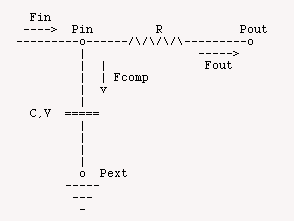## Equations

There are five basic equations which are used in this model. The first equation for flow out of the vessel is related to the resistance by the fluid equivalent of Ohm's Law.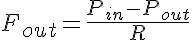where Pout is the pressure at the outlet of the vessel and R is determined from Poiseuille's Law as: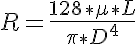where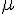is the fluid viscosity, L is the vessel length, and D is the vessel diameter.

The flow into the vessel and the flow out of the vessel are different because of the change in volume which adds or subtracts flow from that leaving the vessel depending on whether the pressure is increasing or decreasing in the vessel. So we have: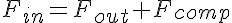where the flow attributed to the vessel compliance, Fcomp, is given by: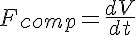and where V is the vessel volume and is related to the compliance, C and intraluminal pressure, Pin, by: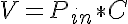In this simulation the initial vessel volume is prescribed by Pin and C is calculated from the initial diameter and vessel length.

The equations for this model may be viewed by running the JSim model applet and clicking on the Source tab at the bottom left of JSim's Run Time graphical user interface. The equations are written in JSim's Mathematical Modeling Language (MML). See the Introduction to MML and the MML Reference Manual. Additional documentation for MML can be found by using the search option at the Physiome home page.

References

Ohm GS. Die galvanische Kette mathematisch bearbeitet, 1827

Key terms
compliant
vessel
flow
resistance
compliance
RC circuit
Acknowledgements

Please cite https://www.imagwiki.nibib.nih.gov/physiome in any publication for which this software is used and send one reprint to the address given below:
The National Simulation Resource, Director J. B. Bassingthwaighte, Department of Bioengineering, University of Washington, Seattle WA 98195-5061.

Model development and archiving support at https://www.imagwiki.nibib.nih.gov/physiome provided by the following grants: NIH U01HL122199 Analyzing the Cardiac Power Grid, 09/15/2015 - 05/31/2020, NIH/NIBIB BE08407 Software Integration, JSim and SBW 6/1/09-5/31/13; NIH/NHLBI T15 HL88516-01 Modeling for Heart, Lung and Blood: From Cell to Organ, 4/1/07-3/31/11; NSF BES-0506477 Adaptive Multi-Scale Model Simulation, 8/15/05-7/31/08; NIH/NHLBI R01 HL073598 Core 3: 3D Imaging and Computer Modeling of the Respiratory Tract, 9/1/04-8/31/09; as well as prior support from NIH/NCRR P41 RR01243 Simulation Resource in Circulatory Mass Transport and Exchange, 12/1/1980-11/30/01 and NIH/NIBIB R01 EB001973 JSim: A Simulation Analysis Platform, 3/1/02-2/28/07.